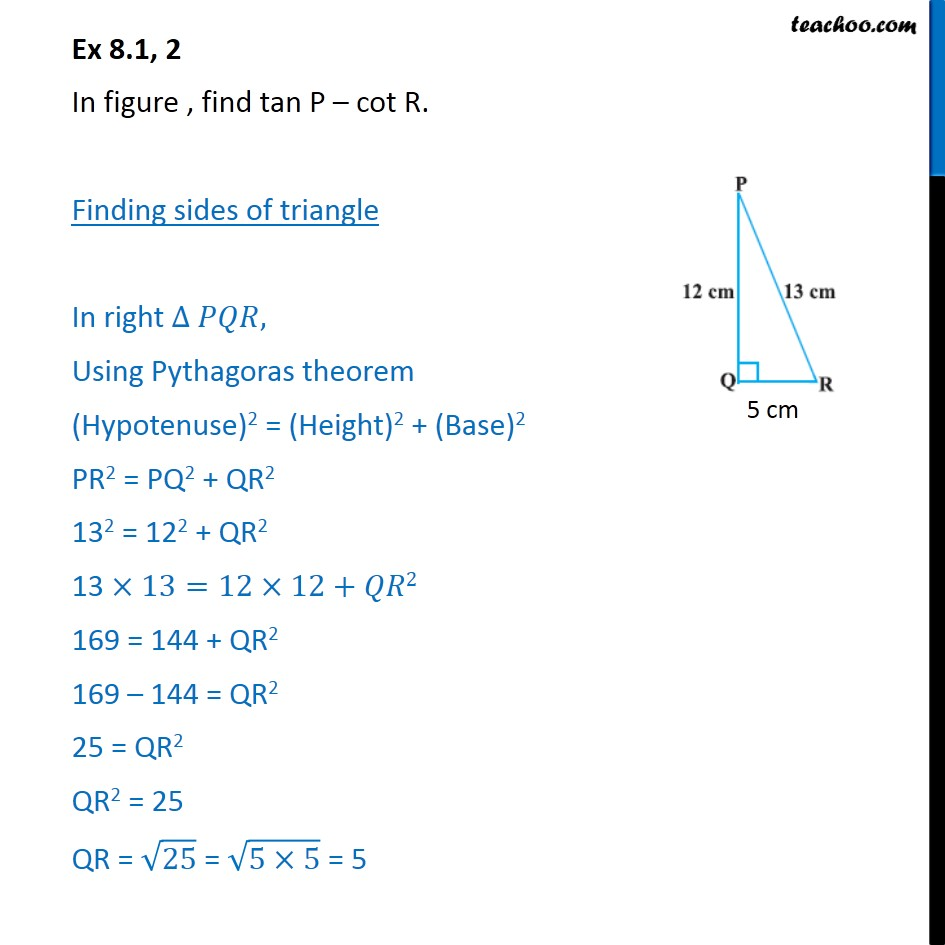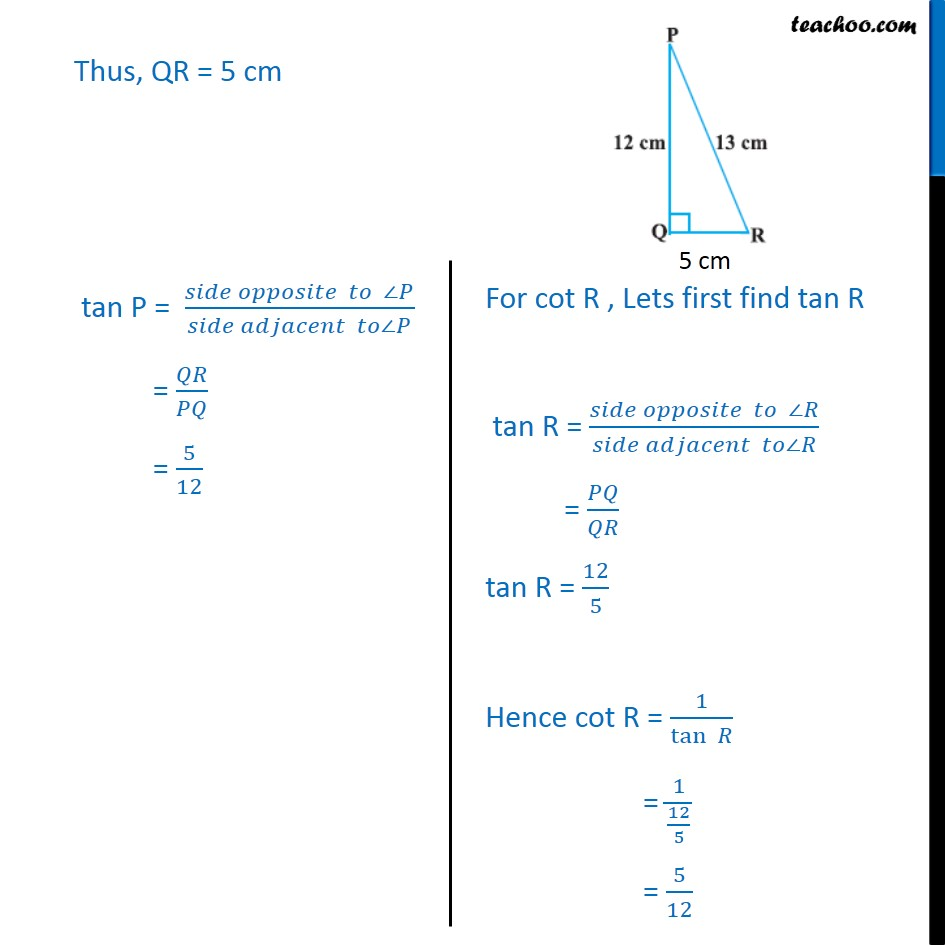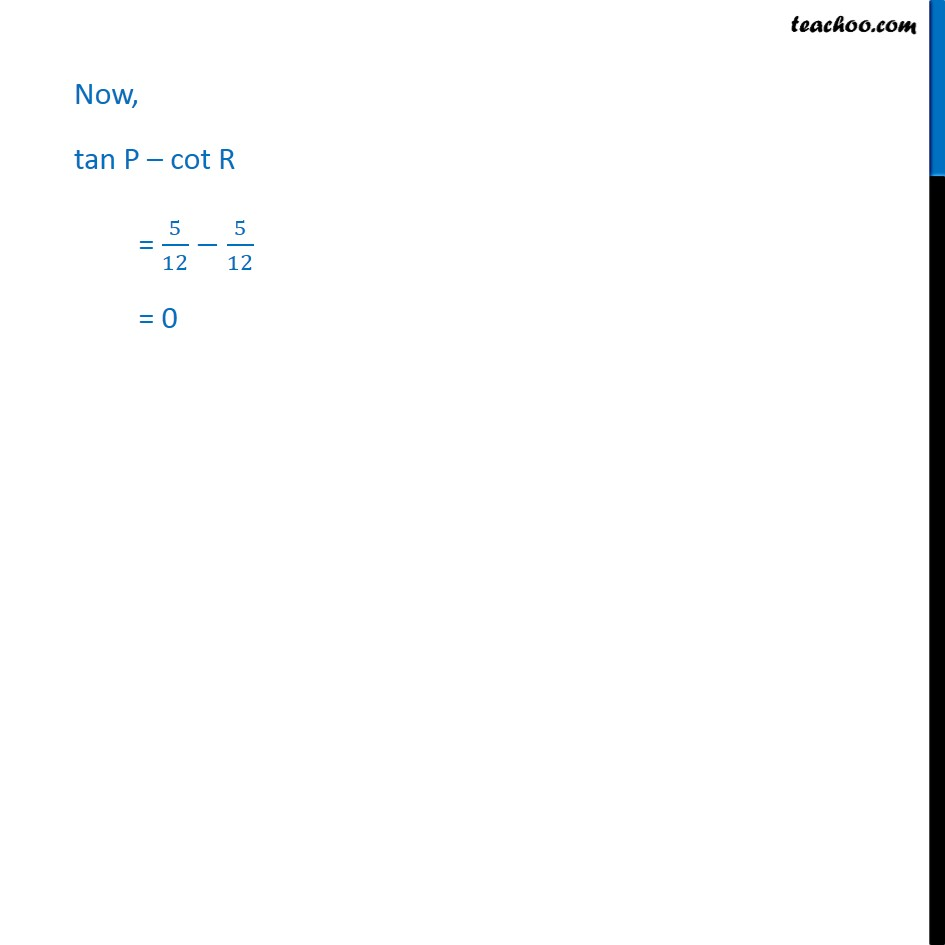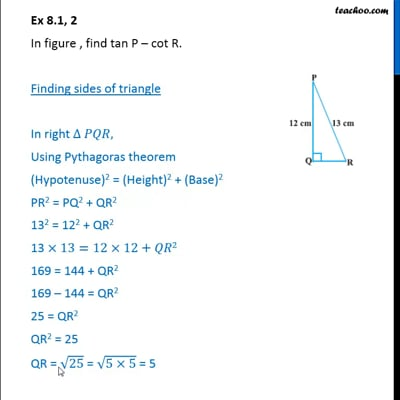Ex 8.1

Chapter 8 Class 10 Introduction to Trignometry
Serial order wiseThis video is only available for Teachoo black users

Solve all your doubts with Teachoo Black (new monthly pack available now!)

### Transcript

Ex 8.1, 2 In figure , find tan P cot R. Finding sides of triangle In right , Using Pythagoras theorem (Hypotenuse)2 = (Height)2 + (Base)2 PR2 = PQ2 + QR2 132 = 122 + QR2 13 13=12 12+ 2 169 = 144 + QR2 169 144 = QR2 25 = QR2 QR2 = 25 QR = 25 = (5 5) = 5 Thus, QR = 5 cm tan P = ( )/( ) = / = 5/12 Now, tan P cot R = 5/12 5/12 = 0